Next: Current Density 4-Vector Up: Relativity and Electromagnetism Previous: Proper Time

# 4-Velocity and 4-Acceleration

We have seen that the quantity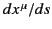transforms as a 4-vector under a general Lorentz transformation [see Equation (1686)]. Because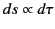it follows that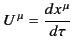(1721)

also transforms as a 4-vector. This quantity is known as the 4-velocity. Likewise, the quantity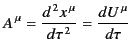(1722)

is a 4-vector, and is called the 4-acceleration.

For events along the world-line of a particle traveling with 3-velocity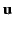, we have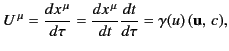(1723)

where use has been made of Equation (1719). This gives the relationship between a particle's 3-velocity and its 4-velocity. The relationship between the 3-acceleration and the 4-acceleration is less straightforward. We have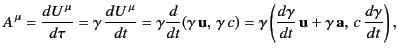(1724)

where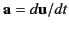is the 3-acceleration. In the rest frame of the particle,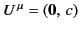and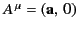. It follows that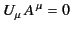(1725)

(note that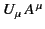is an invariant quantity). In other words, the 4-acceleration of a particle is always orthogonal to its 4-velocity.Next: Current Density 4-Vector Up: Relativity and Electromagnetism Previous: Proper Time
Richard Fitzpatrick 2014-06-27